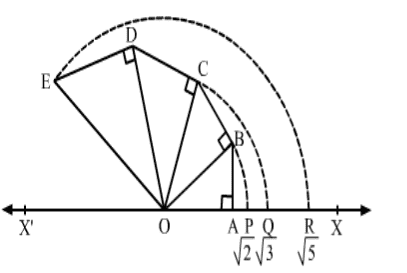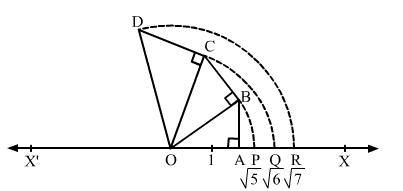# RS Aggarwal Class 9 Solutions Chapter 1 - Real Numbers Ex 1A (1.1)

## RS Aggarwal Class 9 Chapter 1 - Real Numbers Ex 1A (1.1) Solutions Free PDF

The RS Aggarwal Class 9 Solutions prove to be of great value for students looking to score good marks in the exam. These solutions have been prepared by highly experienced subject experts in accordance to the CBSE syllabus of Class 9 maths. It helps in clearing the doubts and understanding each topic in a proper way. Students should refer to these solutions to check whether they have solved the exercise questions correctly so that they don’t repeat the mistake in the exam.

The solutions are presented in an easy way, which makes it easier for the students to read and learn explanations in a quick time. By referring to the RS Aggarwal Class 9 Solutions Chapter 1 Real Numbers Ex 1A (1.1) you can give your preparation the extra edge that is supposed to require in Class 10 board exam.

## RS Aggarwal Class 9 Ex 1A Chapter 1

Question 1: What are irrational numbers? How do they differ from rational numbers? Give examples.

Solution:

A number that can neither be expressed as a terminating decimal nor be expressed as a repeating decimal is called an irrational number. A rational number, on the other hand is always a terminating decimal, and if not, it is a repeating decimal.

Examples of irrational numbers:

0.101001000..

0.232332333..

Question 2: Classify the following numbers as rational or irrational. Give reasons to support your SOLUTION.

(i) 4$\sqrt{4}$

(ii) 196$\sqrt{196}$

(iii) 21$\sqrt{21}$ = 3$\sqrt{3}$ x 7$\sqrt{7}$

(iv) 43$\sqrt{43}$

(v) 3 + 3$\sqrt{3}$

(vi) 7$\sqrt{7}$ – 2

(vii) 23$\frac{2}{3}$ 6$\sqrt{6}$

(viii) .66666666

(ix) 1.232332333…

(x) 3.040040004…

(xi) 3.2576

(xii) 2.356565656…

(xiii) π$\pi$

(xiv) 227$\frac{22}{7}$

Solution:

(i) 4$\sqrt{4}$ = 2

It is a rational number.

(ii) 196$\sqrt{196}$ = 14

It is a rational number.

(iii) 21$\sqrt{21}$ = 3$\sqrt{3}$ x 7$\sqrt{7}$ = 4.58257…

It is an irrational number.

(iv) 43$\sqrt{43}$

If a is a positive integer, which is not a perfect square, then a$\sqrt{a}$ is an irrational number.

Here, 43 is not a perfect square,  so it is irrational.

(v) 3 + 3$\sqrt{3}$

The sum of a rational number and an irrational number is an irrational number.

So, it is an irrational number.

(vi) 7$\sqrt{7}$ – 2

The difference of an irrational number and a rational number is an irrational number, so it is an irrational number.

(vii) 23$\frac{2}{3}$ 6$\sqrt{6}$

The product of a rational number and an irrational number is an irrational number, so it is an irrational number.

(viii) .66666666

It is a rational number because it is a repeating decimal.

(ix) 1.232332333…

It is an irrational number because it is a non-terminating, non- repeating decimal.

(x) 3.040040004…

It is an irrational number because it is a non- terminating, non- repeating decimal.

(xi) 3.2576

It is a rational number because it is a terminating decimal.

(xii) 2.356565656…

It is a rational number because it is repeating.

(xiii) π$\pi$ = 3.14285…

It is an irrational number because it is a non – terminating, non – repeating decimal.

(xiv) 227$\frac{22}{7}$ is a rational number because it can be expressed in the pq$\frac{p}{q}$ form.

Question 3: Represent 2$\sqrt{2}$ , 3$\sqrt{3}$ and 5$\sqrt{5}$ on the real line .

Solution:Let X’ OX be a horizontal line taken as the x – axis and O be the origin representing 0.

Take OA = 1 unit and AB $\perp$ OA such that AB = 1 unit.

Join OB

Now,

OB = OA2+AB2=12+12=2units$\sqrt{OA^{2}+AB^{2}}=\sqrt{1^{2}+1^{2}}=\sqrt{2} units$

Taking O as the center and OB as the radius, draw an arc, meeting OX at P

We have:

OP = OB = 2$\sqrt{2}$ units

Thus, point P represents 2$\sqrt{2}$ on the number line.

Now, draw BC $\perp$ OB such that BC = 1 unit.

Join OC

We have:

OC = OB2+BC2=(2)2+12$\sqrt{OB^{2}+BC^{2}}=\sqrt{(\sqrt{2})^{2}+1^{2}}$ = 3$\sqrt{3}$ units.

Taking O as the centre and OC as the radius, draw an arc, meeting OX at Q.

We have:

OQ = OC = 3$\sqrt{3}$ units.

Thus, point Q represents 3$\sqrt{3}$ on the number line.

Now, draw CD $\perp$ OC such that CD = 1 unit.

Join OD

We have:

OD = OC2+OD2=(3)2+12$\sqrt{OC^{2}+OD^{2}}=\sqrt{(\sqrt{3})^{2}+1^{2}}$ = 4$\sqrt{4}$ = 2 units.

Now, draw DE $\perp$ OD such that DE = 1 unit.

Join OE.

We have:

OD2+DE2=22+12$\sqrt{OD^{2}+DE^{2}}=\sqrt{2^{2}+1^{2}}$ = 5$\sqrt{5}$ units.

Taking O as the center and OE as the radius, draw an arc, meeting OX at R.

We have:

OR = OE = 5$\sqrt{5}$ units

Thus, point R represents 5$\sqrt{5}$ on the number line.

Question 4: Represent 6$\sqrt{6}$ , 7$\sqrt{7}$ and 5$\sqrt{5}$ on the real line .

Solution:Let X’OX be a horizontal line taken as the x – axis and O be the origin representing 0.

Take OA = 2 units and AB $\perp$ OA such that AB = 1 unit.

Now join OB.

We have: OB = OA2+AB2=22+12$\sqrt{OA^{2}+AB^{2}}=\sqrt{2^{2}+1^{2}}$ = 5$\sqrt{5}$ Units

Taking O as the center and OB as the radius, draw an arc, meeting OX at P .

Thus, we have:

OP = OB = 5$\sqrt{5}$ units

Here, point P represents 5$\sqrt{5}$ on the number line.

Now, draw BC $\perp$ OB such that BC = 1 unit.

Join OC

We have: OC = OB2+BC2=(5)2+12=6$\sqrt{OB^{2}+BC^{2}}=\sqrt{(\sqrt{5})^{2}+1^{2}} = \sqrt{6}$ units.

Taking O as the centre and OC as the radius, draw an arc, meeting OX at Q.

Thus, we have:

OQ = OC = 6$\sqrt{6}$ units

Here, point Q represents 6$\sqrt{6}$ on the number line.

Now, draw CD $\perp$ OC such that CD = 1 unit.

Join OD.

We have: OD = OC2+OD2=(6)2+12=7$\sqrt{OC^{2}+OD^{2}}=\sqrt{(\sqrt{6})^{2}+1^{2}} = \sqrt{7}$ units.

Taking O as the centre and OE as the radius, draw an arc, meeting OX at R.

Now,

OR = OD  = 7$\sqrt{7}$

Thus, point R represents 7$\sqrt{7}$ on the number line.

Question 5: Giving reason in each case, show that each of the following members is irrational.

1. 4 + 5$\sqrt{5}$
2. – 3 + 6$\sqrt{6}$
3. 57$5\sqrt{7}$
4. 38$-3\sqrt{8}$
5. 25$\frac{2}{\sqrt{5}}$
6. 43$\frac{4}{\sqrt{3}}$

Solution:

(i)  4 + 5$\sqrt{5}$ because the sum of a rational number and an irrational number is an irrational number.

(ii) – 3 + 6$\sqrt{6}$ because the difference of a rational number and an irrational number is an irrational number.

(iii) 57$5\sqrt{7}$ because the product of a rational number and an irrational number is an irrational number.

(iv) 38$-3\sqrt{8}$ because the product of a rational number and an irrational number is an irrational number.

(v) 25$\frac{2}{\sqrt{5}}$ because the quotient of a rational number and an irrational number is an irrational number.

(vi) 43$\frac{4}{\sqrt{3}}$ because the quotient of a rational number and an irrational number is an irrational number.

Question 6: State in each case, whether the given statement is true or false.

1. The sum of two rational number is rational.
2. The sum of two irrational number is irrational.
3. The product of two rational number is rational.
4. The product of two irrational numbers is irrational.
5. The sum of a rational number and irrational number is irrational.
6. The product of rational number and irrational number is a rational number.
7. Every real number is rational.
8. Every real number is rational or irrational.
9. π$\pi$ is irrational and 227$\frac{22}{7}$ is rational.

Solution:

(i) True

(ii) False

Example: ( 2 + 3$\sqrt{3}$) + (2 – 3$\sqrt{3}$ ) = 4

Here, 4 is a rational number.

(iii) True

(iv) False

Example: 3$\sqrt{3}$ x 3$\sqrt{3}$ = 3

Here, 3 is a rational number.

(v) True

(vi) False

Example: (4) x 5$\sqrt{5}$ = 45$4\sqrt{5}$

Here, 45$4\sqrt{5}$ is an irrational number.

(vii) False

Real numbers can be divided into rational and irrational numbers.

(viii) True

(ix) True

(i) 23$\sqrt{3}$ – 52$\sqrt{2}$ and 3$\sqrt{3}$ + 22$\sqrt{2}$

(ii) 22$\sqrt{2}$ + 53$\sqrt{3}$ – 75$\sqrt{5}$ and 33$\sqrt{3}$2$\sqrt{2}$ + 5$\sqrt{5}$

(iii) 237$\frac{2}{3}\sqrt{7}$122$\frac{1}{2}\sqrt{2}$ +611$\sqrt{11}$  and 137$\frac{1}{3}\sqrt{7}$ + 322$\frac{3}{2}\sqrt{2}$11$\sqrt{11}$

Solution:

(i) 23$\sqrt{3}$ – 52$\sqrt{2}$ + 3$\sqrt{3}$ + 22$\sqrt{2}$

= (23$\sqrt{3}$ + 3$\sqrt{3}$) + (22$\sqrt{2}$ – 52$\sqrt{2}$)

= 33$\sqrt{3}$ – 32$\sqrt{2}$

(ii) 22$\sqrt{2}$ + 53$\sqrt{3}$ – 75$\sqrt{5}$ + 33$\sqrt{3}$2$\sqrt{2}$ + 5$\sqrt{5}$

= 22$\sqrt{2}$2$\sqrt{2}$ + 53$\sqrt{3}$ + 33$\sqrt{3}$ + 5$\sqrt{5}$ – 75$\sqrt{5}$

= 2$\sqrt{2}$ + 83$\sqrt{3}$ – 65$\sqrt{5}$

(iii) 237$\frac{2}{3}\sqrt{7}$122$\frac{1}{2}\sqrt{2}$ +611$\sqrt{11}$  + 137$\frac{1}{3}\sqrt{7}$ + 322$\frac{3}{2}\sqrt{2}$11$\sqrt{11}$

= 237$\frac{2}{3}\sqrt{7}$ + 137$\frac{1}{3}\sqrt{7}$  – 11$\sqrt{11}$ + 611$\sqrt{11}$ + 322$\frac{3}{2}\sqrt{2}$122$\frac{1}{2}\sqrt{2}$

= 7$\sqrt{7}$ + 511$\sqrt{11}$ + 2$\sqrt{2}$

Question 8: MULTIPLY;

1. 35$\sqrt{5}$ by 2 5$\sqrt{5}$
2. 6 15$\sqrt{15}$ by 4 3$\sqrt{3}$
3. 2 6$\sqrt{6}$ by 3 3$\sqrt{3}$
4. 3 8$\sqrt{8}$ by 3 2$\sqrt{2}$
5. 10$\sqrt{10}$ by 40$\sqrt{40}$
6. 3 28$\sqrt{28}$ by 2 7$\sqrt{7}$

Solution:

(i) 35$\sqrt{5}$ x 2 5$\sqrt{5}$ = 3 x 2 x 5$\sqrt{5}$ x 5$\sqrt{5}$ = 6 x 5 = 30

(ii) 6 15$\sqrt{15}$ x 4 3$\sqrt{3}$ = 6 x 4 x 5$\sqrt{5}$ x 3$\sqrt{3}$ x 3$\sqrt{3}$ = 24 x 3 x 5$\sqrt{5}$ = 72 5$\sqrt{5}$

(iii) 2 6$\sqrt{6}$ x 3 3$\sqrt{3}$ = 2 x 3 x 2$\sqrt{2}$ x 3$\sqrt{3}$ x 3$\sqrt{3}$ = 6 x 3 x 2$\sqrt{2}$ = 182$\sqrt{2}$

(iv) 3 8$\sqrt{8}$ x 3 2$\sqrt{2}$ = 3 x 3 x 2$\sqrt{2}$ x 2$\sqrt{2}$ x 2$\sqrt{2}$ x 2$\sqrt{2}$ = 9 x 4 = 36

(v) 10$\sqrt{10}$ x 40$\sqrt{40}$ = 2$\sqrt{2}$ x 5$\sqrt{5}$ x 2$\sqrt{2}$ x 2$\sqrt{2}$ x 2$\sqrt{2}$ x 5$\sqrt{5}$ = 2$\sqrt{2}$ x 2$\sqrt{2}$ x 2$\sqrt{2}$ x 2$\sqrt{2}$ x 5$\sqrt{5}$ x 5$\sqrt{5}$ = 2 x 2 x 5 = 20

(vi) 3 28$\sqrt{28}$ x 2 7$\sqrt{7}$ = 6 7×4$\sqrt{7\times 4}$ x 7$\sqrt{7}$ = 6 x 7 x 4$\sqrt{4}$ = 42 x 2 = 84

Question 9: DIVIDE ;

1. 166,by42${16\sqrt{6}}, by \,{4\sqrt{2}}$
2. 166,by42${16\sqrt{6}}, by \,{4\sqrt{2}}$
3. 166,by42${16\sqrt{6}}, by \,{4\sqrt{2}}$

Solution:

(i) 16642$\frac{16\sqrt{6}}{4\sqrt{2}}$ = 162342$\frac{16\sqrt{2}\sqrt{3}}{4\sqrt{2}}$ = 4 3$\sqrt{3}$

(ii) 16642$\frac{16\sqrt{6}}{4\sqrt{2}}$ = 125×343$\frac{12\sqrt{5}\times \sqrt{3}}{4\sqrt{3}}$ = 3 5$\sqrt{5}$

(iii) 16642$\frac{16\sqrt{6}}{4\sqrt{2}}$ = 187367$\frac{18\sqrt{7}\sqrt{3}}{6\sqrt{7}}$ = 3 3$\sqrt{3}$

### Key Features of RS Aggarwal Class 9 Solutions Chapter 1– Real Numbers Ex 1A (1.1)

• It improves your performance, speed and accuracy.
• All the solutions are explained in a simple language.
• It teaches different techniques to solve tricky and lengthy questions.
• Practicing these solutions will boost up your confidence.

#### Practise This Question

The polynomial ax3+bx2+x6 has (x+2) as a factor and leaves a remainder 4 when divided by (x2). Find a and b.